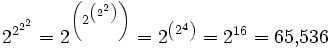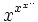A# power tower

A power tower is a way of representing very large numbers in terms of stacks of exponents. For exampleIn general, and especially if the number at the top of the tower is fairly large, adding another exponent to the bottom of a tower will make the value of the tower much larger than will increasing the size of the bottom exponent. This leads to the (somewhat counterintuitive) result that to know which of two towers is the larger, you can look at how many exponents are in the tower and know right away which is larger.

In the case of an infinite power tower of the formthe maximum value that x can take and still cause the tower to converge to a finite value is e(1/e) = 1.444667... The minimum value of x that will produce convergence is 1/ee = 0.065988 ...